## Example Questions

← Previous 1 3 4 5 6 7 8 9 10

### Example Question #2505 : Act Math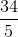Change to a mixed number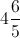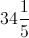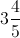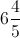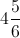Explanation:

To convert from a fraction to a mixed number we must find out how many times the denominator goes into the numerator using division and the remainder becomes the new fraction.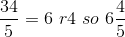### Example Question #2506 : Act Math

What is the average ofand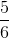?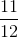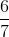Explanation:

To average, we have to add the values and divide by two. To do this we need to find a common denomenator of 6. We then add and divide by 2, yielding 4.5/6. This reduces to 3/4.

### Example Question #2507 : Act Math

Which of the following is equivalent to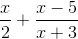?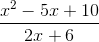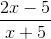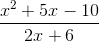None of the answers are correct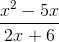Explanation:

This problem is solved the same way ½ + 1/3 is solved.  For example,  ½ + 1/3 = 3/6 + 2/6 = 5/6.  Find a common denominator then convert each fraction into an equivalent fraction using that common denominator.  The final step is to add the two new fractions and simplify.

### Example Question #1 : How To Simplify A Fraction

A train travels at a constant rate ofmeters per second. How many kilometers does it travel inminutes?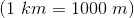Explanation:

Set up the conversions as fractions and solve: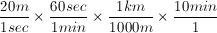### Example Question #3 : How To Simplify A Fraction

Simplify.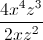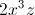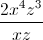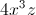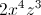Can't be simplifiedExplanation:

To simplify exponents which are being divided, subtract the exponents on the bottom from exponents on the top.  Remember that only exponents with the same bases can be simplified

### Example Question #4 : How To Simplify A Fraction

Simplify: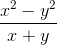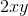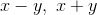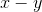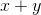Explanation:

x2 – y2 can be also expressed as (x + y)(x – y).

Therefore, the fraction now can be re-written as (x + y)(x – y)/(x + y).

This simplifies to (x – y).

### Example Question #1 : Algebraic Fractions

Simplify: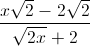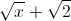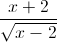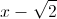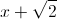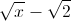Explanation:

Notice that the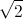term appears frequently. Let's try to factor that out: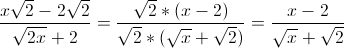Now multiply both the numerator and denominator by the conjugate of the denominator: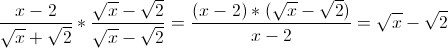### Example Question #6 : How To Simplify A Fraction

Simplify:

(2x + 4)/(x + 2)

2x + 2

x + 1

x + 4

x + 2

2

2

Explanation:

(2x + 4)/(x + 2)

To simplify you must first factor the top polynomial to 2(x + 2). You may then eliminate the identical (x + 2) from the top and bottom leaving 2.

### Example Question #7 : How To Simplify A Fraction

Simplify the following expression: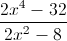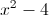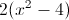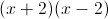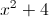Explanation:

Factor both the numerator and the denominator: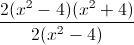After reducing the fraction, all that remains is: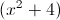### Example Question #8 : How To Simplify A Fraction

Simplify: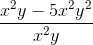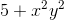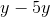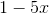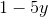Explanation:

With this problem the first thing to do is cancel out variables. The x2 can all be divided by each other because they are present in each system. The equation will now look like this: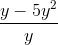Now we can see that the equation can all be divided by y, leaving the answer to be:← Previous 1 3 4 5 6 7 8 9 10

### All ACT Math Resources